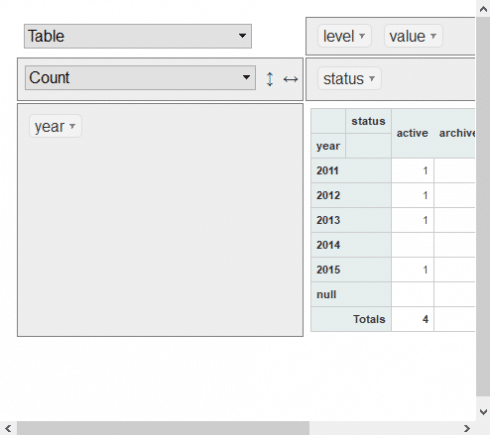# Using Data Cubes with R

May 16, 2019
By

(This article was first published on R Programming – DataScience+, and kindly contributed to R-bloggers)

Interested in guest posting? We would love to share your codes and ideas with our community.

## Tags

Data cubes are a popular way to display multidimensional data. This makes the method suitable for big data. Giving the incredible growth of data it is natural that the method have become increasingly popular. In this article you learn to use R for data cubes.

## Read data cubes pacakages into R

First we will read the packages into the R library:

```# Read packages into R library
library(data.table)
library(data.cube)
library(rpivotTable)
```

Next we will build a sample array for the data cube:

```# Crerate sample slide array
set.seed(1L)
ar.dimnames = list(color = sort(c("green","yellow","red")),
year = as.character(2011:2015),
status = sort(c("active","inactive","archived","removed")))
ar.dim = sapply(ar.dimnames, length)
ar = array(sample(c(rep(NA, 4), 4:7/2), prod(ar.dim), TRUE),
unname(ar.dim),
ar.dimnames)
print(ar)

cb = as.cube(ar)
print(cb)
str(cb)
all.equal(ar, as.array(cb))
all.equal(dim(ar), dim(cb))
all.equal(dimnames(ar), dimnames(cb))

print(cb)

fact:
fact 30 rows x 4 cols (0.00 MB)
dims:
color 3 rows x 1 cols (0.00 MB)
year 5 rows x 1 cols (0.00 MB)
status 4 rows x 1 cols (0.00 MB)
total size: 0.01 MB
> str(cb)
Classes 'cube', 'R6'.
cube\$env\$fact: List of 1
\$ fact:Classes ‘data.table’ and 'data.frame':	30 obs. of  4 variables:
cube\$env\$dims: List of 3
\$ color :Classes ‘data.table’ and 'data.frame':	3 obs. of  1 variable:
\$ year  :Classes ‘data.table’ and 'data.frame':	5 obs. of  1 variable:
\$ status:Classes ‘data.table’ and 'data.frame':	4 obs. of  1 variable:

```

Now it is time to create a slice and dice for the data cube:

```# slice

arr = ar["green",,]
print(arr)
r = cb["green",]
print(r)
all.equal(arr, as.array(r))

arr = ar["green",,,drop=FALSE]
print(arr)
r = cb["green",,,drop=FALSE]
print(r)
all.equal(arr, as.array(r))

arr = ar["green",,"active"]
r = cb["green",,"active"]
all.equal(arr, as.array(r))

# dice

arr = ar["green",, c("active","archived","inactive")]
r = cb["green",, c("active","archived","inactive")]
all.equal(arr, as.array(r))
as.data.table(r)
as.data.table(r, na.fill = TRUE)

print(arr)
status
year   active archived inactive removed
2011     NA       NA       NA     3.0
2012    3.5       NA       NA     2.5
2013    3.5       NA        3     3.0
2014     NA       NA       NA      NA
2015    2.5       NA        3     2.0
print(r)

fact:
fact 9 rows x 3 cols (0.00 MB)
dims:
year 5 rows x 1 cols (0.00 MB)
status 4 rows x 1 cols (0.00 MB)
total size: 0.01 MB
print(arr)
, , status = active

year
color   2011 2012 2013 2014 2015
green   NA  3.5  3.5   NA  2.5

, , status = archived

year
color   2011 2012 2013 2014 2015
green   NA   NA   NA   NA   NA

, , status = inactive

year
color   2011 2012 2013 2014 2015
green   NA   NA    3   NA    3

, , status = removed

year
color   2011 2012 2013 2014 2015
green    3  2.5    3   NA    2
print(r)

fact:
fact 9 rows x 4 cols (0.00 MB)
dims:
color 1 rows x 1 cols (0.00 MB)
year 5 rows x 1 cols (0.00 MB)
status 4 rows x 1 cols (0.00 MB)
total size: 0.01 MB
> as.data.table(r)
year   status value
1: 2012   active   3.5
2: 2013   active   3.5
3: 2013 inactive   3.0
4: 2015   active   2.5
5: 2015 inactive   3.0
> as.data.table(r, na.fill = TRUE)
year   status value
1: 2011   active    NA
2: 2011 archived    NA
3: 2011 inactive    NA
4: 2012   active   3.5
5: 2012 archived    NA
6: 2012 inactive    NA
7: 2013   active   3.5
8: 2013 archived    NA
9: 2013 inactive   3.0
10: 2014   active    NA
11: 2014 archived    NA
12: 2014 inactive    NA
13: 2015   active   2.5
14: 2015 archived    NA
15: 2015 inactive   3.0

```

Now it is time to make apply rollup and drilldown for the data cube

```# apply
format(aggregate(cb, c("year","status"), sum))
format(capply(cb, c("year","status"), sum))

# rollup and drilldown
# granular data with all totals
r = rollup(cb, MARGIN = c("color","year"), FUN = sum)
format(r)

# chose subtotals - drilldown to required levels of aggregates
r = rollup(cb, MARGIN = c("color","year"), INDEX = 1:2, FUN = sum)
format(r)
```

Now lets try to make the data cube into a pivottable:

```# pivot
r = capply(cb, c("year","status"), sum)
format(r, dcast = TRUE, formula = year ~ status)
library(rpivotTable)
r = rollup(cb, c("year","status"), FUN = sum, normalize=FALSE)
rpivotTable(r,rows="year", cols=c("status"),width="100%", height="400px")
```

This gives us the following pivottable in html:### References

Related Post

R-bloggers.com offers daily e-mail updates about R news and tutorials on topics such as: Data science, Big Data, R jobs, visualization (ggplot2, Boxplots, maps, animation), programming (RStudio, Sweave, LaTeX, SQL, Eclipse, git, hadoop, Web Scraping) statistics (regression, PCA, time series, trading) and more...